# ChatGPT and spreadsheets: is generating formulas a thing of the past?Written by
Marcos MurataChatGPT has taken the world by storm and has become the fastest-growing web platform of all time. Within two months, this AI-powered chatbot developed by OpenAI reached 100 million users all over the world.

Hundreds of millions of users are using ChatGPT to write essays, translate texts, solve math problems and even write code.

Since everybody is jumping on the ChatGPT bandwagon, we’ve decided to test this artificial-intelligence tool to understand if it can help us manipulate data and create formulas in Google Sheets.

Can ChatGPT help spreadsheet users create formulas effortlessly?

### How good is ChatGPT at creating Google Sheet formulas?

In order to answer this question, we decided to ask ChatGTP “how good is ChatGTP at generating Google Sheet formulas?”. Here’s the answer: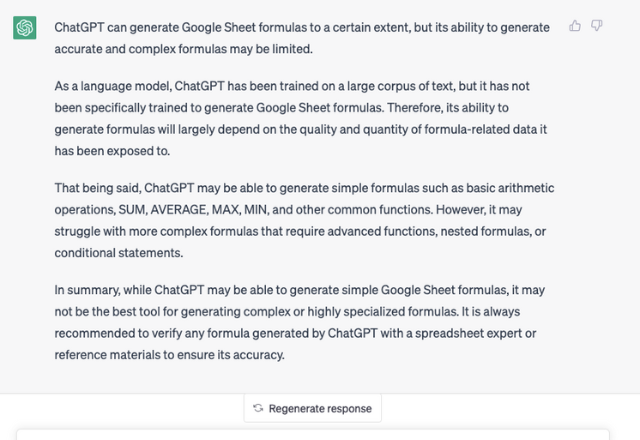ChatGPT can generate Google Sheet formulas to a certain extent, but its ability to generate accurate and complex formulas may be limited.

As a language model, ChatGPT has been trained on a large corpus of text, but it has not been specifically trained to generate Google Sheet formulas. Therefore, its ability to generate formulas will largely depend on the quality and quantity of formula-related data it has been exposed to.

That being said, ChatGPT may be able to generate simple formulas such as basic arithmetic operations, SUM, AVERAGE, MAX, MIN, and other common functions. However, it may struggle with more complex formulas that require advanced functions, nested formulas, or conditional statements.

In summary, while ChatGPT may be able to generate simple Google Sheet formulas, it may not be the best tool for generating complex or highly specialized formulas. It is always recommended to verify any formula generated by ChatGPT with a spreadsheet expert or reference materials to ensure its accuracy.

It seems that ChatGPT can create simple formulas but it may struggle to generate more complex formulas. However, we’re not going to take ChatGPT’s word for it.

Let’s put ChatGPT to the test and find out how well it can generate formulas in Google Sheets.

### First challenge: creating an array formula with an if function

Generate a formula that calculates the difference between a date in column B and today in days, unless column D is filled, in which case it should calculate the difference between the date in column B and the date in column D. Use it inside an ARRAYFORMULA().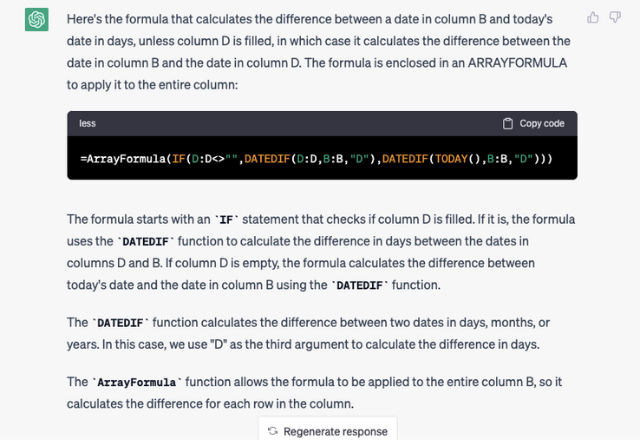Here’s the formula that calculates the difference between a date in column B and today’s date in days, unless column D is filled, in which case it calculates the difference between the date in column B and the date in column D. The formula is enclosed in an ARRAYFORMULA to apply it to the entire column:

=ArrayFormula(IF(D:D<>””,DATEDIF(D:D,B:B,”D”),DATEDIF(TODAY(),B:B,”D”)))

The formula starts with an IF statement that checks if column D is filled. If it is, the formula uses the DATEDIF function to calculate the difference in days between the dates in columns D and B. If column D is empty, the formula calculates the difference between today’s date and the date in column B using the DATEDIF function.

The DATEDIF function calculates the difference between two dates in days, months, or years. In this case, we use “D” as the third argument to calculate the difference in days.

The ArrayFormula function allows the formula to be applied to the entire column B, so it calculates the difference for each row in the column.

Although ChatGPT managed to generate a formula from the prompt, the result wasn’t correct. As you can see, when we apply the formula, it returns an error.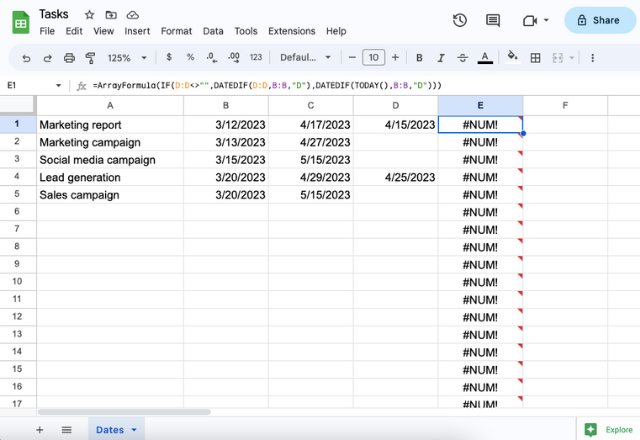### Second challenge: using an array formula with sum

Generate a formula that calculates the difference between a date in column B and today in days. Use it inside an ARRAYFORMULA().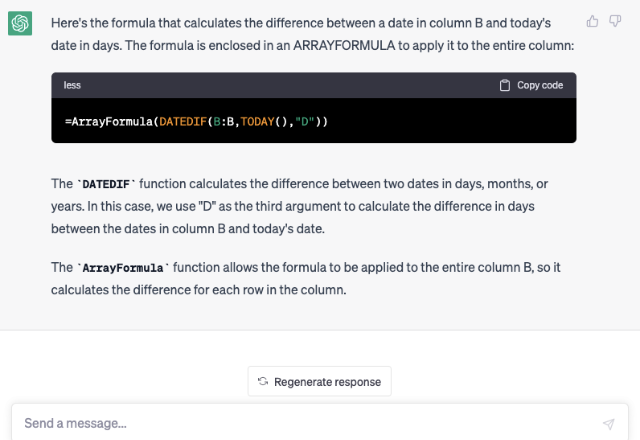Here’s the formula that calculates the difference between a date in column B and today’s date in days. The formula is enclosed in an ARRAYFORMULA to apply it to the entire column:

=ArrayFormula(DATEDIF(B:B,TODAY(),”D”))

The DATEDIF function calculates the difference between two dates in days, months, or years. In this case, we use “D” as the third argument to calculate the difference in days between the dates in column B and today’s date.

The ArrayFormula function allows the formula to be applied to the entire column B, so it calculates the difference for each row in the column.

Now ChatGPT has created the right formula to calculate the difference between a date in a column and today’s date.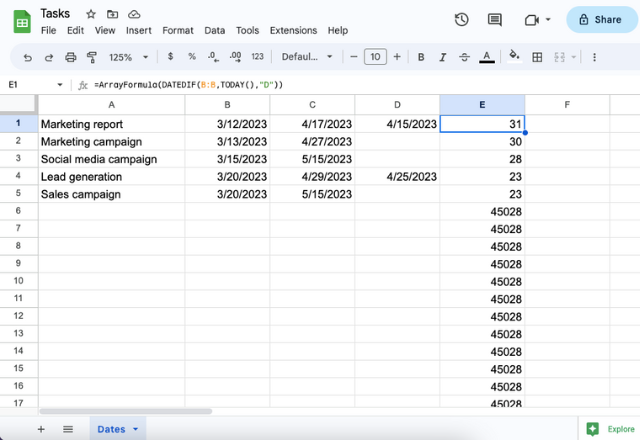### Conclusion

Apparently, ChatGPT’s answer to the question “how good is ChatGTP at generating Google Sheet formulas?” has summed it all up in a few words.

In summary, while ChatGPT may be able to generate simple Google Sheet formulas, it may not be the best tool for generating complex or highly specialized formulas.

If you don’t know how to create simple formulas in Google Sheets, ChatGPT can come in handy. Not only does it generate basic formulas, but it also gives us a brief explanation of how it works.

However, if you need to create more advanced formulas, you may have to wait a little longer. Although ChatGPT has evolved very fast, it is still not ready to alleviate the workload of spreadsheet users who need to generate complex formulas in Google Sheets.

It’s hard to know what the future holds for artificial intelligence. Things are changing, and they are changing very fast. Microsoft has recently announced that it is bringing ChatGPT technology to Word, Excel and Outlook.

At this pace, spreadsheet formulas may soon become a thing of the past.

If you want to leverage the power of AI, check out these awesome ChatGPT prompts for spreadsheet users.